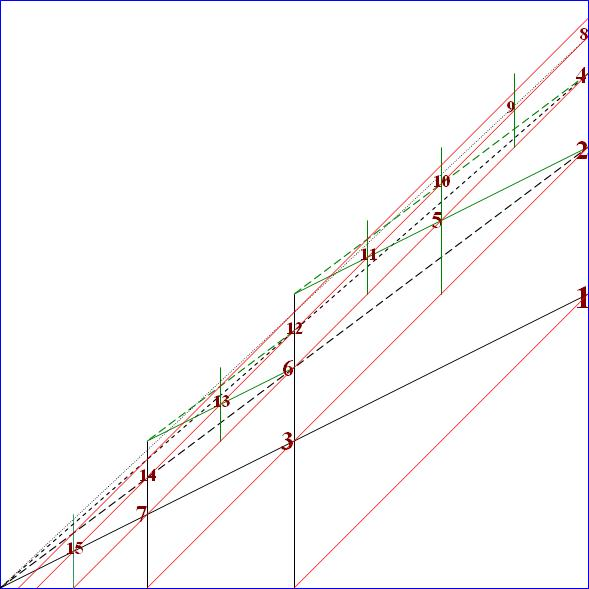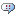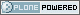# DBAzine.com

##### Personal tools
You are here: Home » Integer Labeling in Nested Intervals Model
Seeking new owner for this high-traffic DBAzine.com site.
##### Who Are You?
I am a:
Mainframe True Believer
Distributed Fast-tracker

[ Results | Polls ]

# Integer Labeling in Nested Intervals Model

This article completes the series of articles exploring Binary Rational labeling for Nested Intervals Model. The advantage of this model is that every node has a permanent label so that insertion of a new node doesn’t affect existing nodes. In this article, we’ll reduce the node labeling to just a single positive integer number.

## Single Number Labeling

The Nested Intervals Model labeled each node with a binary rational number, which is essentially a pair of integer numbers. Given that a set of all pairs of integer numbers can be enumerated, it easily follows that we can code each node with one integer only. This is not a very interesting idea, however. We save some storage, but for all practical querying purposes, we would have to translate into binary rational numbers. Plus, who cares about storage limitations nowadays?

We’ll explore a different approach: employing a triangular navigation method. This method is similar to the enumeration mentioned in the previous paragraph, but we do not refer to numerator and denominator values explicitly. The picture below illustrates the idea:We start with node <x=1,y=1/2>, which we label with 1, move diagonally left down along the “red” line, but find no more nodes. Next, we navigate along the red, diagonally aligned line just above it. Our starting point is <x=1,y=3/4>, which we label with the next integer, 2. Next, we move diagonally left down along the “red” line, find node <x=1/2,y=1/4>, and label it with integer 3. In the following step, we’ll navigate the next red diagonal just above our last one, and label them nodes 4,5,6, and 7.

In other words, numbers at every diagonally aligned line form an arithmetic progression. Hence, we’ll call them arithmetic siblings. Don’t confuse those with real siblings, which are, as you might recall from the introductory article, aligned along the lines that meet at a breadth-first convergence point. The other distinction is that each line drawn through the sibling nodes has a slope less than that of the diagonal.

You might have already noticed that every first (or left-most) child is twice as big as its parent. Therefore, we call all nodes on each vertical line geometric descendants.

An immediate property of the previously mentioned labeling schema is density: all integer positive numbers are utilized. Since some database implementations have integers of limited range only, density might become an attractive feature, because one would be able to store and manipulate bigger trees before hitting the arithmetic overflow exception. (Even though we’e getting a little ahead of ourselves, I’ll mention that this idea didn’t prove to work; please note the conclusion section of the paper for more comments.)

## Partial Order Relation

Single integer labeling allows a very simple definition of the transitive closure relation. Given 2 nodes, i and j, such that i <= j, let k be the maximum integer, satisfying i*2k <= j. For example, if i=1 and j=11, then k=3 and i*2k=8. Geometrically, the i*2k is the intersection of the arithmetic siblings line originated in j and geometric descendants line originated in i.

Consider the binary relation ancestor of satisfying the following condition:

`i ancestor of j <=> j-i*2k < 2k-1`

Informally, we’ve required j to be closer to i*2k than i to k if we adjust for “geometric” distance between i and i*2k. For example, let i=1, j=12, then k=3 and i*2k=8. We have j-i*2k = 4 and 2k-1 = 4, therefore 12 is not an ancestor of 1. Indeed, 12 = 3*22 is an ancestor of node 3, which is a sibling of node 1 (if we are allowed to consider the roots of the forest as siblings).

From the definition itself, that ancestor of a transitive relation is not quite obvious. Our new integer labeling schema, however, has a very simple connection to the Materialized path and Binary Rational Encoding, which makes transitivity obvious.

## Ordered Integer Partitions

For each node labeled with Numer and Denom by Binary Rational Encoding schema, let

`N = Denom - (Numer+1)/4 `

Here is a list of 16 tree nodes in 3 three different encodings:

 N PATH NUMER/DENOM 1 .1 3/2 2 .1.1 7/4 3 .2 3/4 4 .1.1.1 15/8 5 .1.2 11/8 6 .2.1 7/8 7 .3 3/8 8 .1.1.1.1 31/16 9 .1.1.2 27/16 10 .1.2.1 23/16 11 .1.3 19/16 12 .2.1.1 15/16 13 .2.2 11/16 14 .3.1 7/16 15 .4 3/16 16 .1.1.1.1.1 63/32

Notice that the PATH encoding for each node between N=2k-1, and N=2k-1 is an ordered partition of integer k. Also, note that Denom in each partition of k equals 2k. It immediately follows that the sum of dot-separated numbers in any path is the logarithm of Denom.

N is the same encoding that we introduced in previous sections! The relation ancestor of, therefore, is just a reflection of the relation is prefix of defined on Materialized Paths, which is obviously transitive.

## Distance Function

Distance function is a straightforward implementation of ancestor relation defined in the previous section:

```function dist ( child integer, parent integer )RETURN integer IS   ret integer;   arifgeom integer;   pwr integer;BEGIN   if parent > child then      RETURN -1;   end if;   if parent = child then      RETURN 0;   end if;   arifgeom := parent;   pwr := 0;   while arifgeom*2 <= child loop      arifgeom := arifgeom*2;      pwr := pwr+1;   end loop;   if child-arifgeom < arifgeom/(parent*2) then      RETURN pwr;   end if;   RETURN -1;END;
select dist(11,3), dist(12,3), dist(13,3), dist(14,3) from dual-1      2      2      -1```

In theory, distance function is all that is required to answer hierarchical queries. In practice, however, query with distance function implies scanning the whole hierarchy table and applying the ancestor of predicate to every row. With this method, we couldn’t advance beyond small hierarchies.

## Querying Node’s Ancestors

A typical query that doesn’t require scanning the whole hierarchy might ask to find the list of node’s ancestors. Similarly, when a materialized path, nested intervals, and binary rational labelings answer such a query, this doesn’t involve querying the hierarchy relation at all. The Parent label calculation in our new integer labeling schema is simplicity itself. If the child number is even, then child divided by 2 gives us the parent. Otherwise, we won’t jump to the parent right away, but instead, navigate to adjacent (real) sibling with smaller encoding. Technically this means subtracting 1, and dividing the result by 2:

```function immediate_parent ( child integer )RETURN integer IS   ret integer;BEGIN   ret := child;   while mod(ret,2)=1 loop      ret := (ret-1)/2;   end loop;   RETURN ret/2;END;/
select immediate_parent(21) from dual5```

Therefore, queries involving ancestors can be answered efficiently by iterative navigation through the ancestors chain. These iterating calls to the immediate_parent function can be conveniently wrapped inside a table function. (Another common name for the table function is “virtual view”).

If a list of ancestors is used as a part of more complex report, then it would make sense creating index on a node number column (having a primary key on the node number might be a good idea, anyway). Assuming that the list of ancestors is small compared to the total number of rows in the hierarchy table, it would be more efficient to access each individual node on the ancestors list by a unique index scan.

## Mapping to Interval Encoding

Unlike ancestor queries, we can’t leverage the immediate_parent function when querying the node’s descendants. Even if we develop a dual version, immediate_child(child# integer), we still couldn’t plug it into a single query. In this circumstances, the only efficient method I’m aware of is Nested Sets approach. Given a node encoded as an interval [x,y], we qualify all the nodes [x',y'] as the descendants whenever y < x' < x (or, equivalently, y < y' < x). This is typically an index range scan. Therefore, we need to be able to convert our single number labels into the interval.

First, given a node N, we define k as

`k = floor(log(2,N))`

Then, we notice that arithmetic siblings are evenly spaced with horizontal distance 1/2k between neighbor siblings. Therefore, the horizontal distance between nodes N and 2k is (N-2k)/2k. On the other hand, this distance is the same as the difference between x coordinate of node 2k and x coordinate of the node N, that is 1-x. Our first equation tying the interval coordinate x to N follows immediately:

`1-x = (N-2k)/2k`

can be rewritten as

`x = (2k+1-N)/2k`

Next, we leverage the constraint that node N lies on the arithmetic siblings line. Each arithmetic siblings line has a slope of -1, so the equation must have the form

`y-x = const`

Knowing that the line intersects the (x=1, y=1-1/2k+1) point, we refine the line equation to

`y-x = -1/2k+1`

which, after substituting x, can be rewritten as

`y = (2k+2-2*N-1)/2k+1`

When implementing these equations, be aware of the artifacts like limited precision of the logarithm function:

```select log(2,4)-2, floor(log(2,4)) from dual
LOG(2,4)-FLOOR(2) FLOOR(LOG(2,4))----------------- ----------------2.000E-38        1```

Therefore, we can’t rely on floor(log(2,N)), but have to use our homegrown function:

`function floorLog2 ( N integer )RETURN integer IS   k integer;   kpow2 integer;BEGIN   k := 0;   kpow2 := 1;   while kpow2 <= N loop      k := k+1;      kpow2 := kpow2*2;   end loop;   RETURN k-1;END;`

Now that the snag is removed we can proceed:

```function x_num ( N integer )RETURN integer IS   ret_num integer;   ret_den integer;   k       integer;BEGIN   k := floorlog2(N);   ret_num := power(2,k+1)-N;   ret_den := power(2,k);   while mod(ret_num,2)=0       ret_num := ret_num/2;      ret_den := ret_den/2;   end loop;   RETURN ret_num;END;
function x_den ( N integer )RETURN integer IS...   RETURN ret_den;END;
function y_num ( N integer )RETURN integer IS   ret_num integer;   ret_den integer;   k       integer;BEGIN   k := floorlog2(N);   ret_num := power(2,k+2)-2*N-1;   ret_den := power(2,k+1);   while mod(ret_num,2)=0 loop      ret_num := ret_num/2;      ret_den := ret_den/2;   end loop;   RETURN ret_num;END;
function y_den ( N integer )RETURN integer IS...   RETURN ret_den;END;
select path(normalize_numer(numer,denom),            normalize_denom(numer,denom)) from (   select x_num(N)*y_den(N)+y_num(N)*x_den(N) numer,          x_den(N)*y_den(N) denom from (      select rownum N from emp   ));
N PATH-- ---------- 1 .1 2 .1.1 3 .2 4 .1.1.1 5 .1.2 6 .2.1 7 .3 8 .1.1.1.1 9 .1.1.210 .1.2.111 .1.312 .2.1.113 .2.214 .3.1```

In the test above, we have selected a list of nodes numbered from 1 to 14, then calculated interval boundaries x and y. Next, we added x and y together to convert to a single binary rational encoding. Since numerator, as a result of the previous step, can be an even number, we have to normalize the numerator and denominator before submitting them as arguments to the path function.

The final steps would be amending the hierarchy table to leverage new encoding

`create table emps (   node          integer,   employee_name varchar2(30),   salary        number,   ...)`

and replacing the view

`create or replaceview hierarchy as  select node,         y_num(node) numer_left,         y_den(node) denom_left,         x_num(node) numer_right,         x_den(node) denom_right,         integer2path(node) path,         dist(node,1) depth,         employee_name,         salary,         ...   from emps`

Writing integer2path(node) function is left as an exercise for you.

## Conclusion

Even though we are able to encode a tree node with a single integer, we still have to use Binary Rational encoding for query purposes. Unfortunately, denominators in the encoding sequence of all the children of the node “1”

 PATH BINARY ENCODING .1.1 7/4 .1.2 11/8 .1.3 19/16

are growing exponentially. Likewise, the members of a depth-first sequence

 PATH BINARY ENCODING .1 3/2 .1.1 7/4 .1.1.1 15/8

are growing exponentially as well. As of today, databases implement integers of a limited range and, therefore, one won’t be able to store and manipulate trees of any significant size before hitting the arithmetic overflow exception.

This flaw can be fixed by switching to a different encoding. Database developers are welcome to implement a new efficient method proposed in

http://arxiv.org/html/cs.DB/0401014

and

http://arxiv.org/abs/cs.DB/0402051

## Acknowledgments

This is a revision of the paper that was originally submitted to SIGMOD Record. The author thanks the anonymous reviewer who spotted a flaw in the transitivity proof.

--

Vadim Tropashko still works for the Real World Performance group at Oracle Corp.

###Powers of 2

Posted by mikharakiri at 2005-06-24 11:19 AM
After dbazine migrated to the new look-and-feel, the bugs crept into this article. All superscripts are missing: 2k is 2^k (that is 2 power k) in all the formulas.
Transaction Management
Reduce downtime and increase repeat sales by improving end-user experience.
Free White Paper
Database Recovery
Feeling the increased demands on data protection and storage requirements?